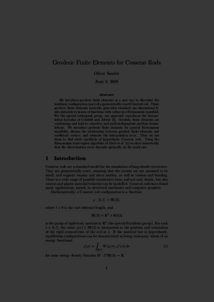Repository: Freie Universität Berlin, Math Department

# Geodesic finite elements for Cosserat rods

Sander, O. (2010) Geodesic finite elements for Cosserat rods. International Journal for Numerical Methods in Engineering, 82 (13). pp. 1645-1670. ISSN 1097-0207Preview

489kB

Official URL: https://dx.doi.org/10.1002/nme.2814

## Abstract

We introduce geodesic finite elements as a new way to discretize the non-linear configuration space of a geometrically exact Cosserat rod. These geodesic finite elements naturally generalize standard one-dimensional finite elements to spaces of functions with values in a Riemannian manifold. For the special orthogonal group, our approach reproduces the interpolation formulas of Crisfield and Jelenić. Geodesic finite elements are conforming and lead to objective and path-independent problem formulations. We introduce geodesic finite elements for general Riemannian manifolds, discuss the relationship between geodesic finite elements and coefficient vectors, and estimate the interpolation error. Then we use them to find static equilibria of hyperelastic Cosserat rods. Using the Riemannian trust-region algorithm of Absil et al. we show numerically that the discretization error depends optimally on the mesh size. Copyright © 2009 John Wiley & Sons, Ltd.

Item Type: Article Mathematical and Computer Sciences > Mathematics > Numerical Analysis Department of Mathematics and Computer Science > Institute of Mathematics 1882 Ekaterina Engel 13 Apr 2016 10:45 03 Mar 2017 14:42

Repository Staff Only: item control page